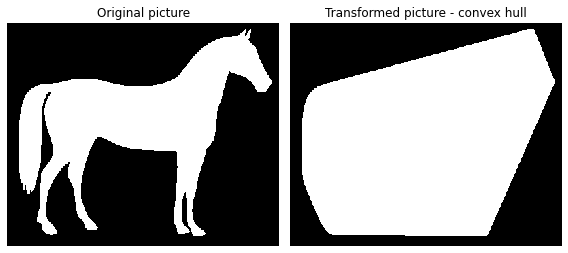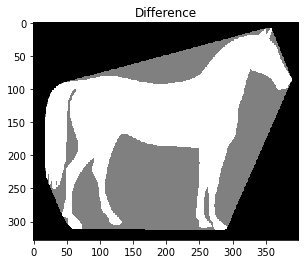# 8. Convex Hull¶

The convex hull of a binary image is the set of pixels included in the smallest convex polygon that surround all white pixels in the input.

A good overview of the algorithm is given on this blog.

import matplotlib.pyplot as plt

from skimage.morphology import convex_hull_image
from skimage import data, img_as_float
from skimage.util import invert


## 8.1. The original image is inverted as the object must be white¶

image = invert(data.horse())


## 8.2. Create a convex hull¶

chull = convex_hull_image(image)


## 8.3. Show the result¶

fig, axes = plt.subplots(1, 2, figsize=(8, 4))
ax = axes.ravel()

ax.set_title('Original picture')
ax.imshow(image, cmap=plt.cm.gray, interpolation='nearest')
ax.set_axis_off()

ax.set_title('Transformed picture - convex hull')
ax.imshow(chull, cmap=plt.cm.gray, interpolation='nearest')
ax.set_axis_off()

plt.tight_layout()
plt.show()## 8.4. Showing the difference by fixing the pixel intensity on the convex hull¶

chull_diff = img_as_float(chull.copy())
chull_diff[image] = 2

fig, ax = plt.subplots()
ax.imshow(chull_diff, cmap=plt.cm.gray, interpolation='nearest')
ax.set_title('Difference')
plt.show()